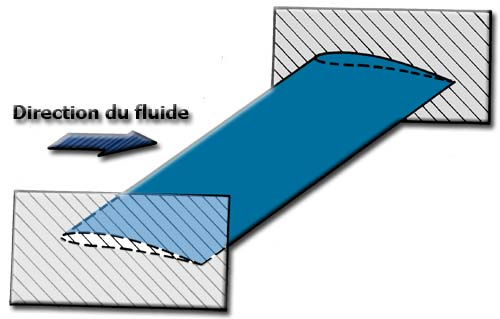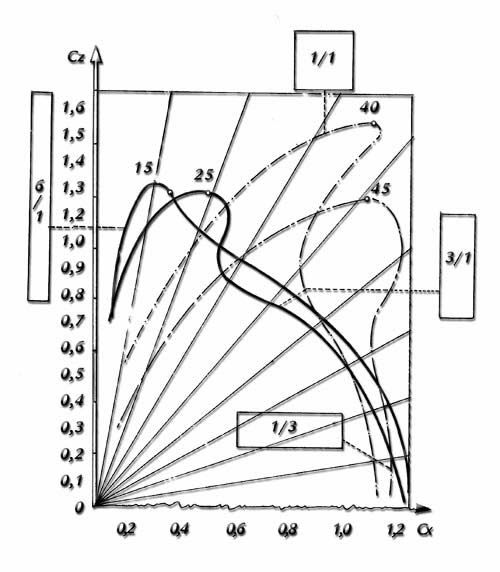Modeling a wing mast sail in heliciel Introducing the mecaflux software suite: Wings or foils modeling in heliciel Aerial propeller modeling in heliciel Boat propeller modeling in heliciel Ventilation propeller modeling in heliciel Wind turbine propeller modeling in heliciel Tidal turbine modeling in heliciel Kaplan propeller modeling in heliciel
Aspect ratio of the blade and aspect ratio of the wing

• Among the geometric parameters of a Wing, One of the most important parameters to characterize a wing is the aspect ratio. This is the ratio between the square of the span , b, and the projection of the surface of the wing, S:

Aspect ratio = AR = b²/S

The aspect ration does not have a unit;

For rectangular wings having a constant chord c along the span for all profiles, S = bc, the aspect ratio is AR = b/c . generally, the values ​​of elongation are 20 to 40 for gliders (or ultralight aircraft) and 6 to 10 for small and medium speed aircraft. The aspect ratio decreases when the speed of the plane becomes larger, such that for supersonic aircraft, the aspect ratio is in the range from 2 to 3. The wings profiles whose performance is given as a coefficient CD,CL...are called aspect ration infinite AR= infinite because performance is given excluding the effects of wing tip losses. When the performance tests from which we extract the coefficients of the profiles are made in the wind tunnel, the wingtips representative profiles are between plates that cancel the effects of loss of the wing tips.Some aircraft have been equipped with measuring system for airfoils, To do this plates frame the profile to simulate a wing of infinite length (span):We say, therefore, that profiles performance are given for an infinite span wing. The term 2D performance is also used to differentiate the 3D performance that take into account the finite nature of the wing or blade. The impact of the aspect ratio in the performance of a wing ar sail or blade of finite length is highlighted by this curve gives us the Eiffel coefficients (3D) lift and drag CD CL for curved plates of different aspect ratios:The quality of a wing is its lift / drag ratio. We note that the plate aspect ratio 6/1 generates the best lift / drag ratio. The plate with the highest aspect ratio who generates the best performance. If we compare the evolution of early wings that men built: Sails, we see the old forms to increase their aspect ratio till today:It is therefore important during the construction of a wing , or a sail or a propeller blade to remember that the aspect ratio is synonym of energy saving. the tip losses calculation allow us to predict the performance 3D of a wing using its profiles 2D performance taking account of its aspect ratio and distribution of vortices which are formed mainly at the blade tip, but influence a large part of the wing performances.

 Rectangular wing length of 2 meters Aspect ratio = 4 ratio (Lift / Drag) =16 Rectangular wing length of 9 meters Aspect ratio = 18 ratio (Lift / Drag) =32 Triangular wing length of 2 meters. Aspect ratio = 5 ratio (Lift / Drag) =19. Triangular wing length of 9 meters. Aspect ratio = 33 ratio (Lift / Drag) =36

To learn more:Theory wing of finite span based on the method of distribution of vortices.